# Multiplying DecimalsPage 1

#### WATCH ALL SLIDES

Slide 1## Multiplying Decimals

Slide 2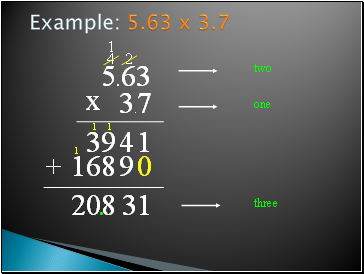Example: 5.63 x 3.7

5.63

3.7

x

1

2

4

4

39

0

9

8

1

16

+

1

3

1

8

1

0

1

2

two

one

three

.

Slide 3## To Multiply

You do not line up the factors by the decimal.

Instead, place the number with more digits on top.

Line up the other number underneath, at the right.

Multiply

Count the number of decimal places (from the right) in each factor.

Use the total number of decimal places in your two factors to place the decimal in your product.

Slide 4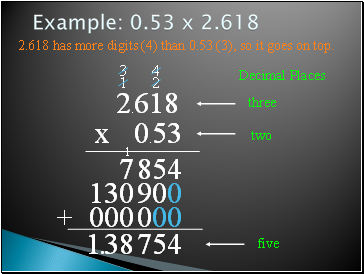Example: 0.53 x 2.618

2.618 has more digits (4) than 0.53 (3), so it goes on top.

2.618

0.53

x

4

2

5

8

1

7

0

0

4

9

0

3

13

00

0

0

0

0

+

4

5

7

1

8

3

1

Decimal Places

three

two

five

.

Slide 5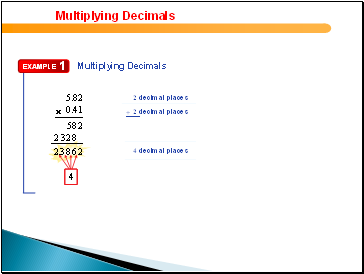## Multiplying Decimals

5.82

0.41

2 decimal places

2 decimal places

5 8 2

2 3 2 8

4 decimal places

2.3 8 6 2

–––––––

1

2

3

4

Slide 6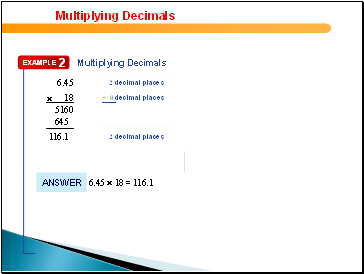6.45

18

2 decimal places

0 decimal places

5160

645

––––––

116.10

Multiplying Decimals

2 decimal places

6.45  18 = 116.1

Slide 76.45

18

2 decimal places

0 decimal places

5160

645

––––––

116.10

1.273

0.06

3 decimal places

2 decimal places

7638

0.0

5 decimal places

Multiplying Decimals

2 decimal places

Slide 8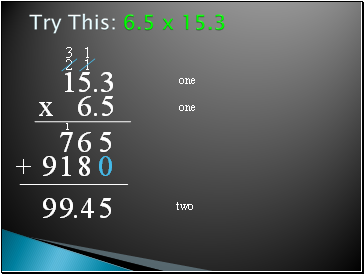Try This: 6.5 x 15.3

15.3

6.5

x

5

1

6

2

7

0

8

1

1

3

9

+

5

4

1

9

9

one

one

two

.

Slide 9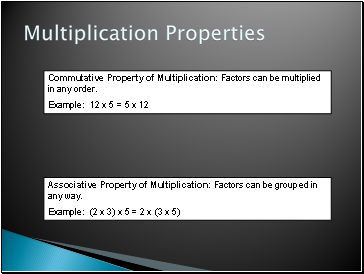## Multiplication Properties

Commutative Property of Multiplication: Factors can be multiplied in any order.

Example: 12 x 5 = 5 x 12

Associative Property of Multiplication: Factors can be grouped in any way.

Example: (2 x 3) x 5 = 2 x (3 x 5)

Slide 10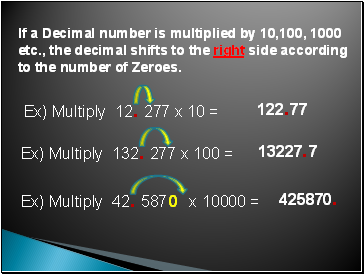If a Decimal number is multiplied by 10,100, 1000 etc., the decimal shifts to the right side according to the number of Zeroes.

Go to page:
1  2